# RD Sharma Solutions for Class 11 Maths Chapter 2 Relations

## RD Sharma Solutions Class 11 Maths Chapter 2 – Free PDF Available

RD Sharma Solutions for Class 11 Maths Chapter 2 – Relations are available here. In the previous chapter, we have discussed various operations on sets to create more sets out of given sets. In this chapter, we shall study one more operation which is known as the cartesian product of sets. This will finally enable us to introduce the concept of relation. Students having any doubts in understanding the concepts can refer to RD Sharma Solutions Class 11 Maths.

The solutions are created by subject matter experts having vast experience in the academic field. Pdf consisting of RD Sharma Solutions Class 11 Maths Chapter 2 are available here, which can be easily downloaded from the links provided below.

Chapter 2- Relations contains three exercises and the RD Sharma Class 11 Solutions present in this page provide solutions to the questions present in each exercise. Now, let us have a look at the concepts discussed in this chapter.

• Ordered pairs.
• Cartesian product of sets.
• Graphical representation of the cartesian product of sets.
• The number of elements in the cartesian product of two sets.
• Diagramatic representation of the cartesian product of two sets.
• Relations.
• Representation of a relation.
• Domain and range of a relation.
• The inverse of a relation.

## Download the Pdf of RD Sharma Solutions for Class 11 Maths Chapter 2 – Relations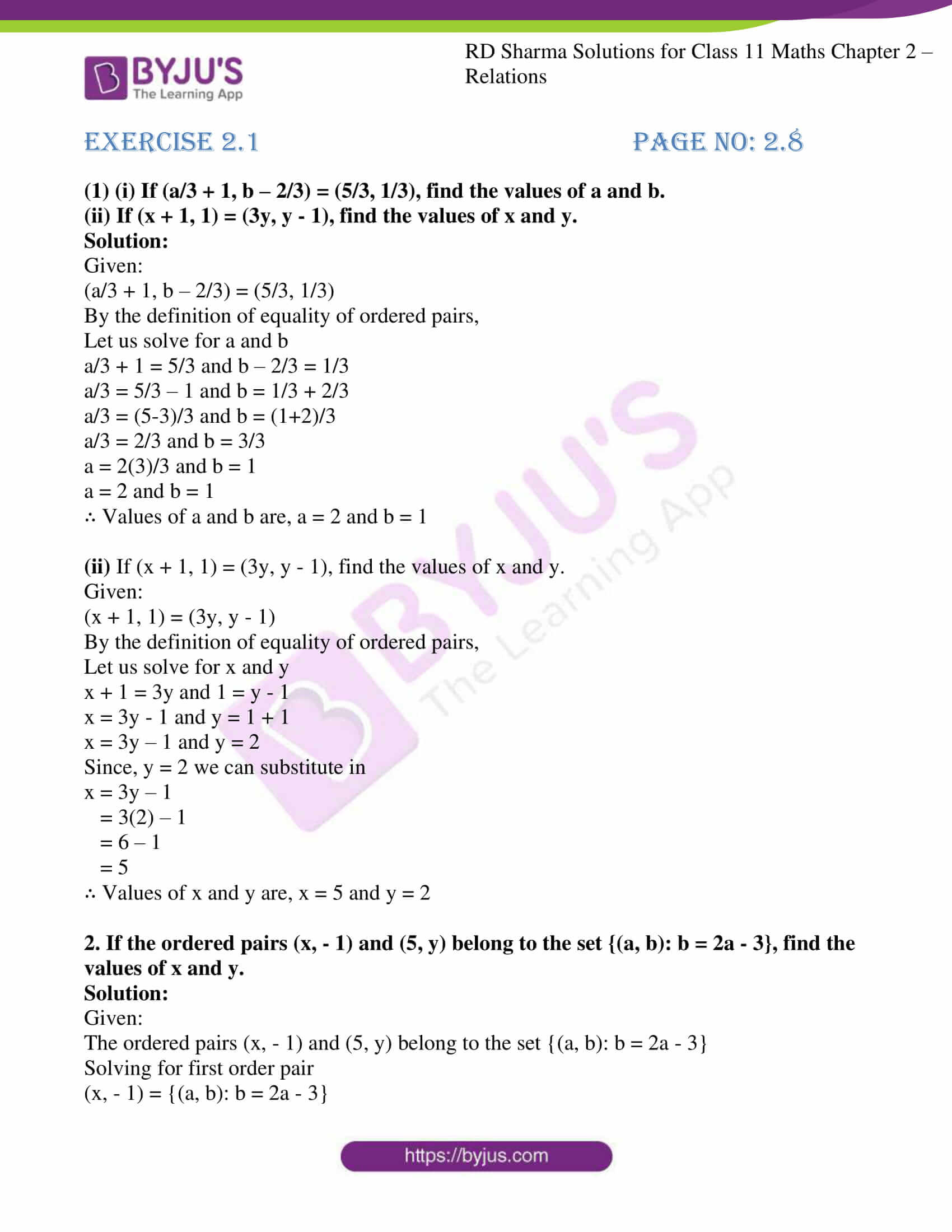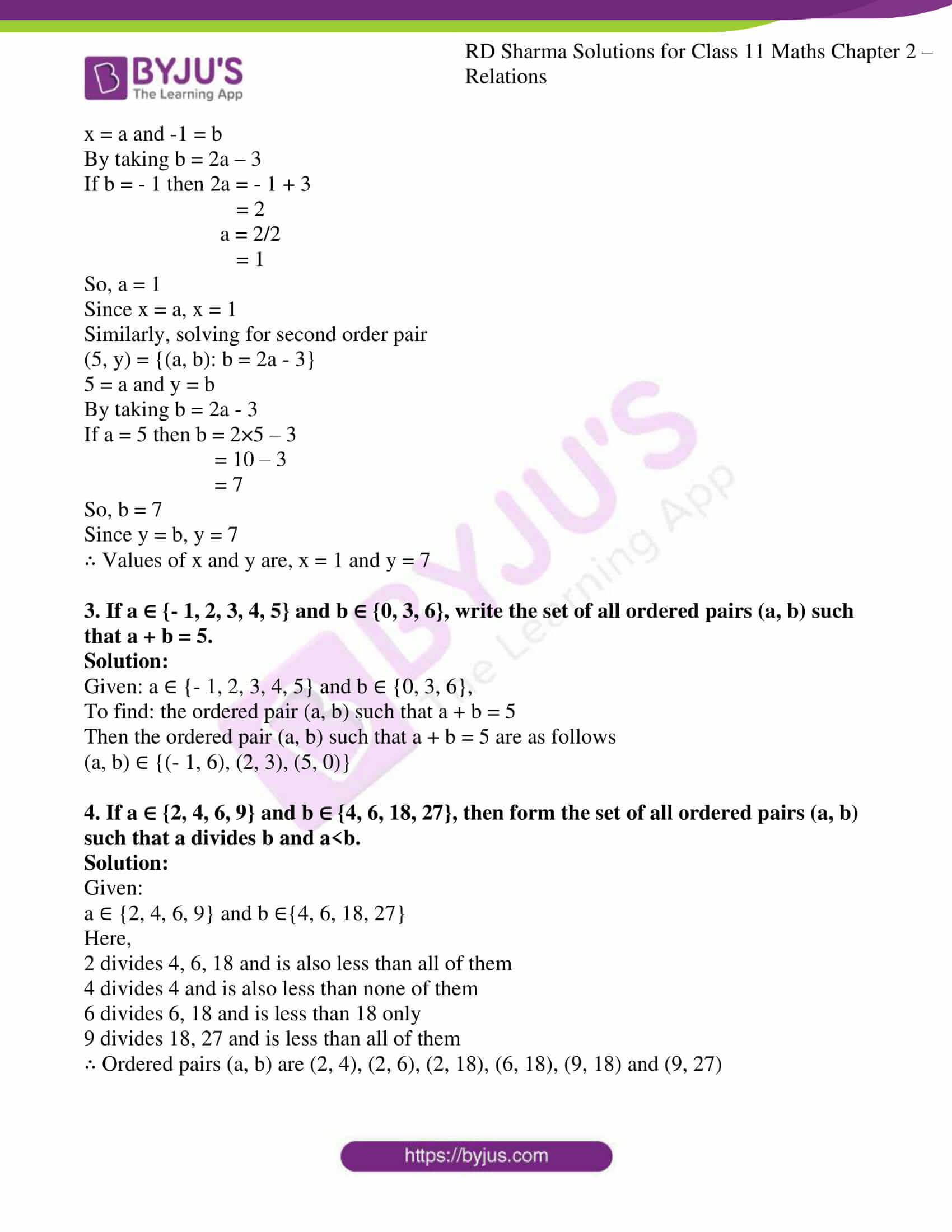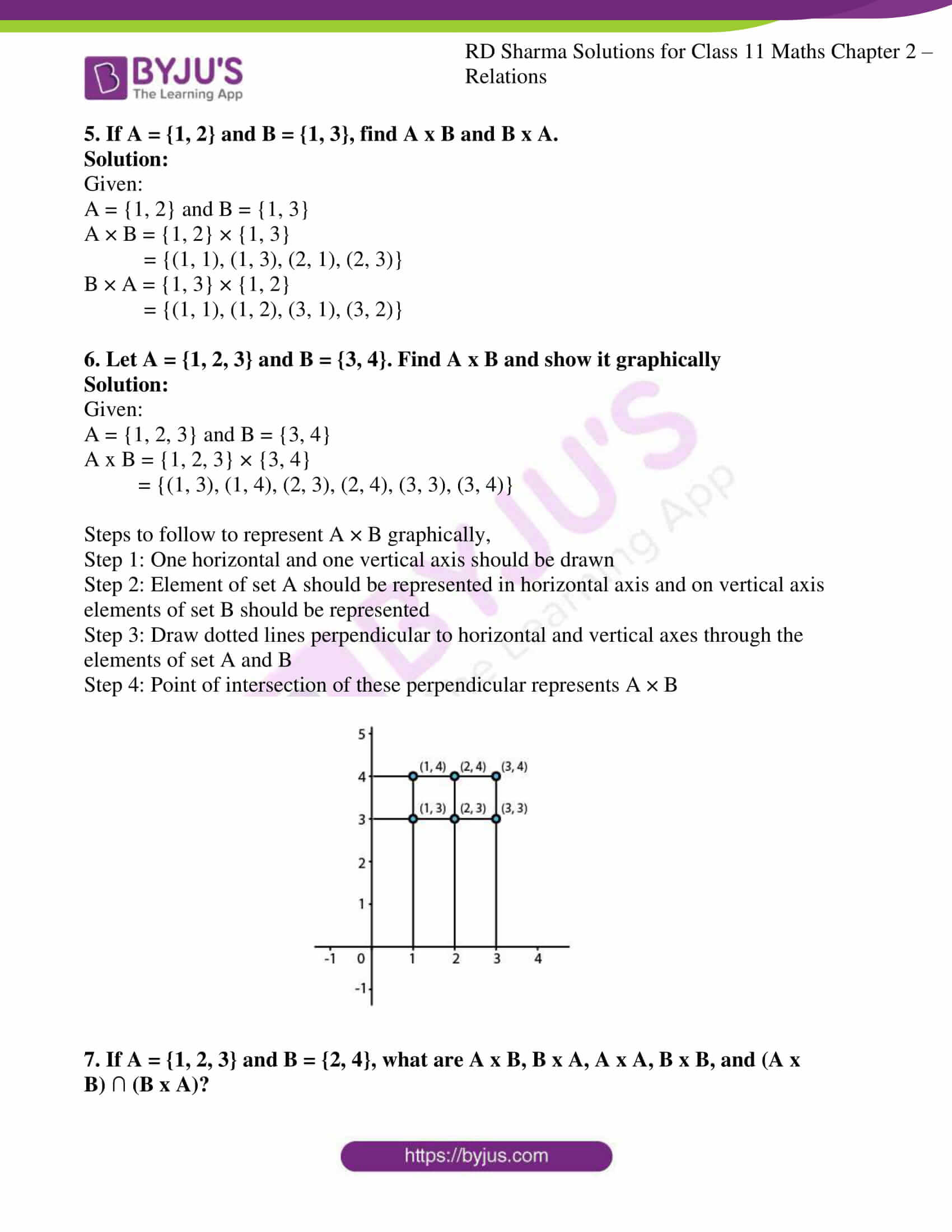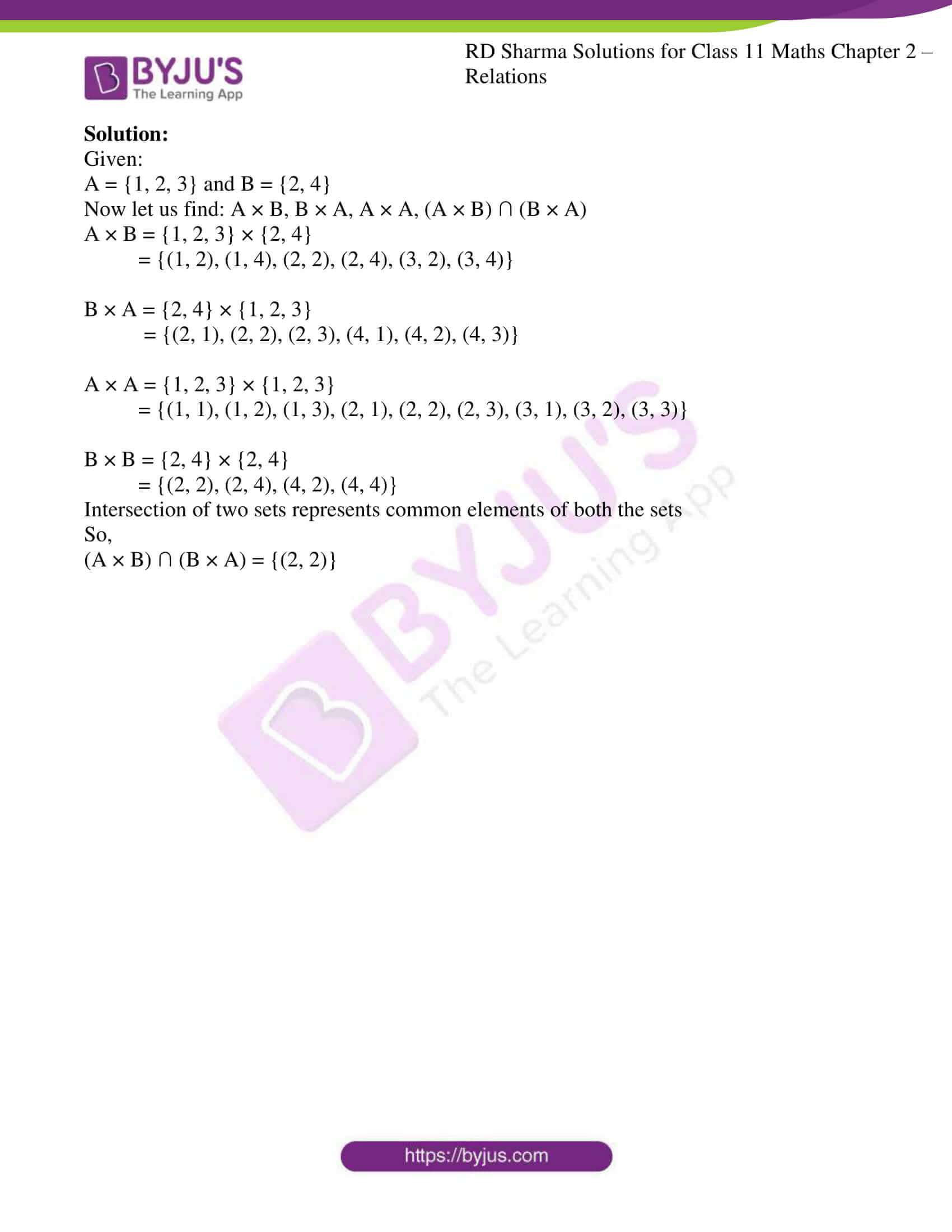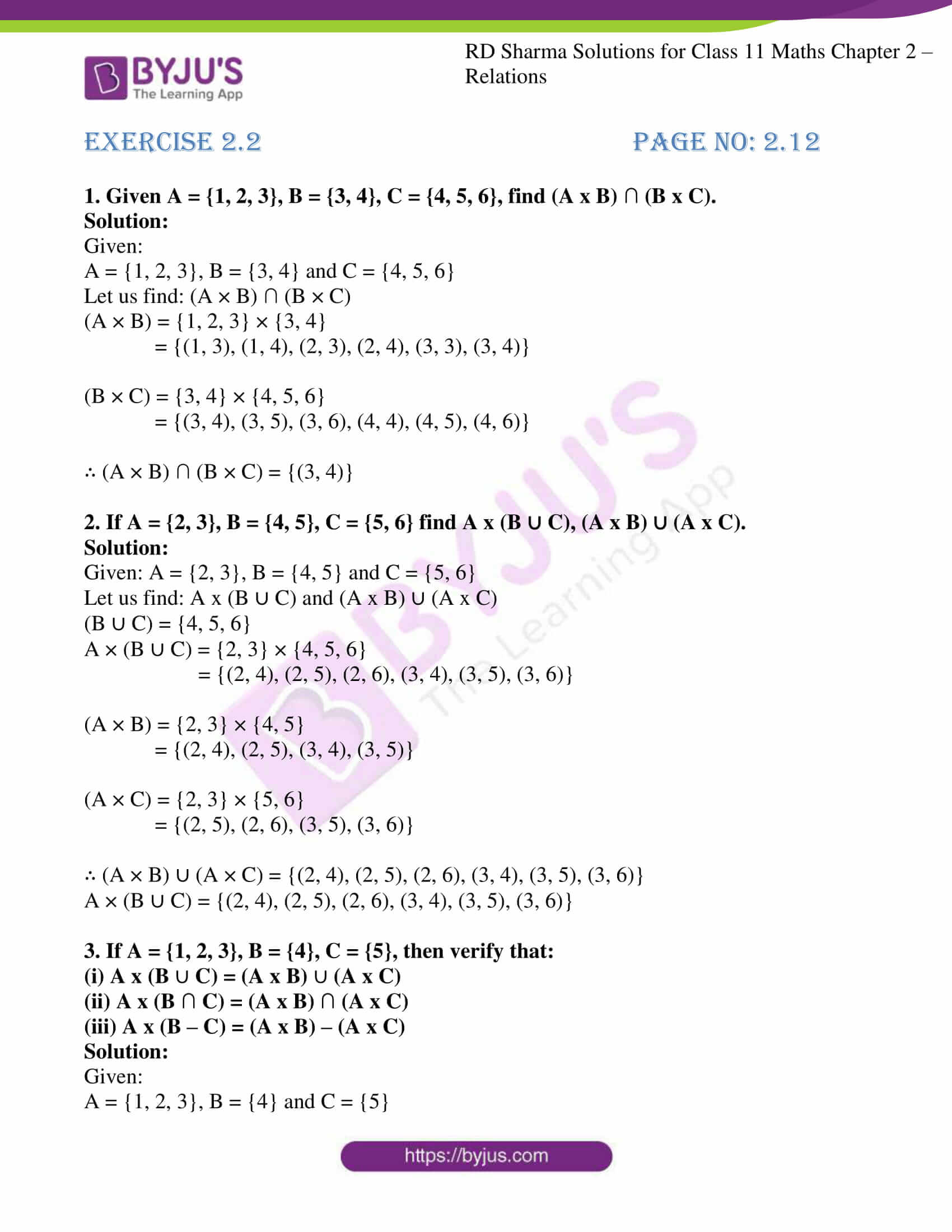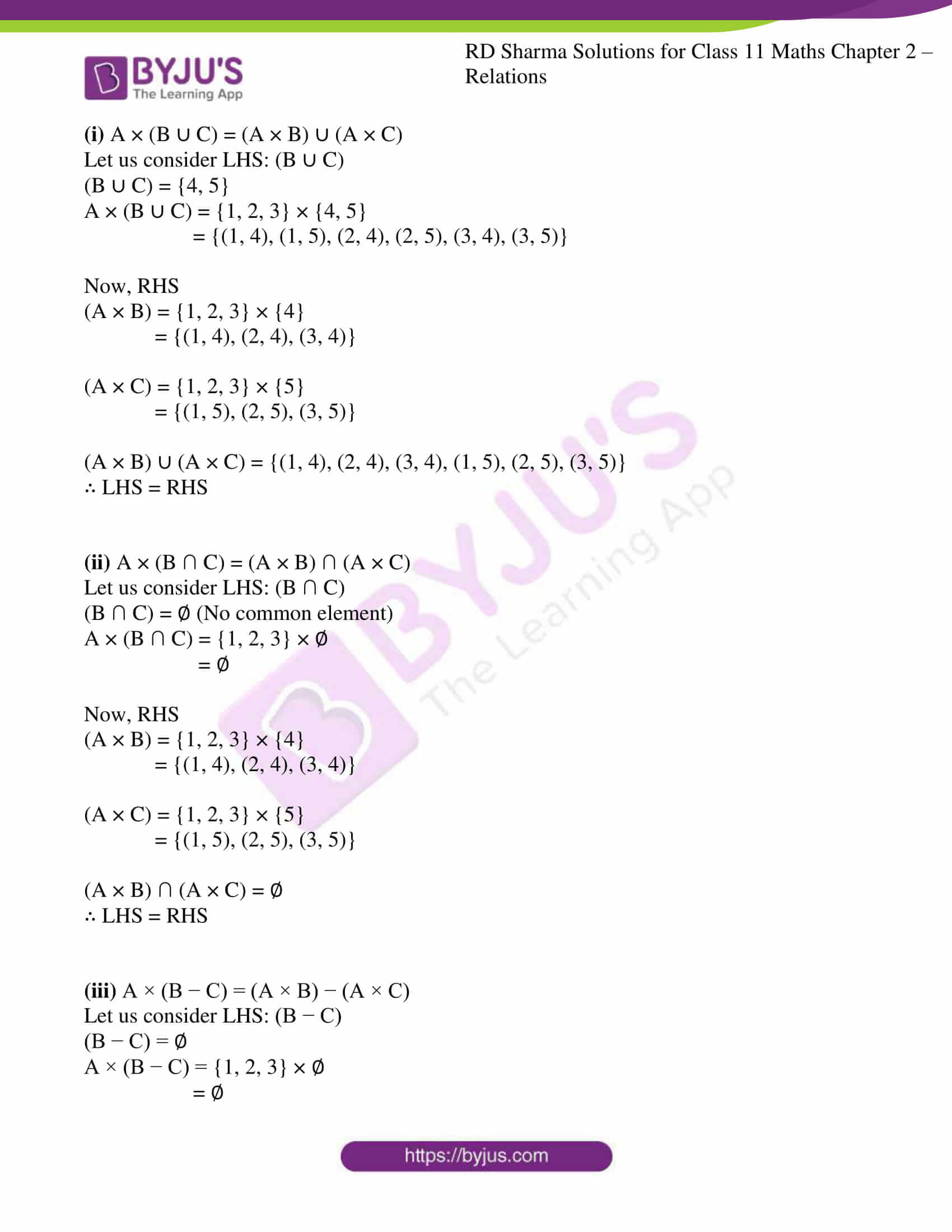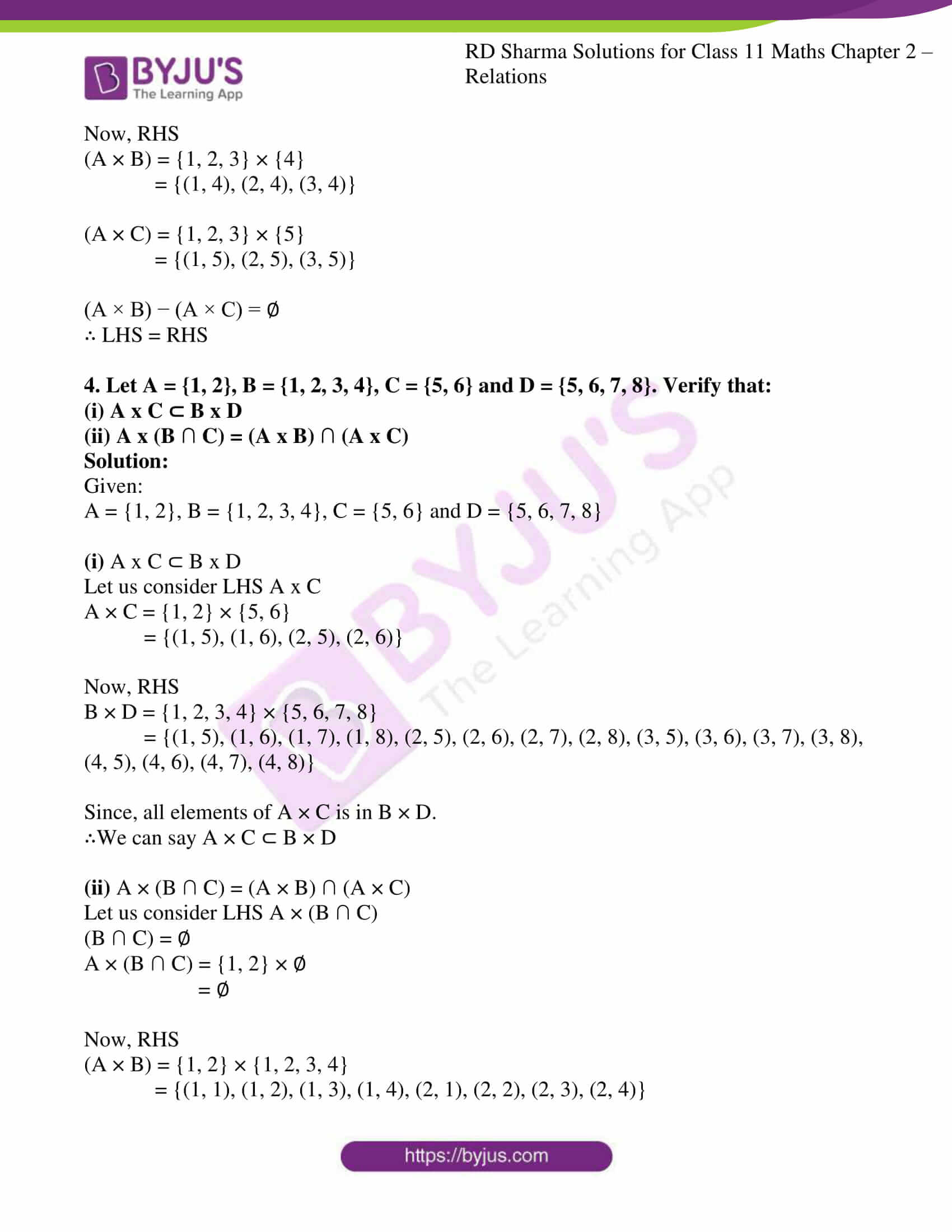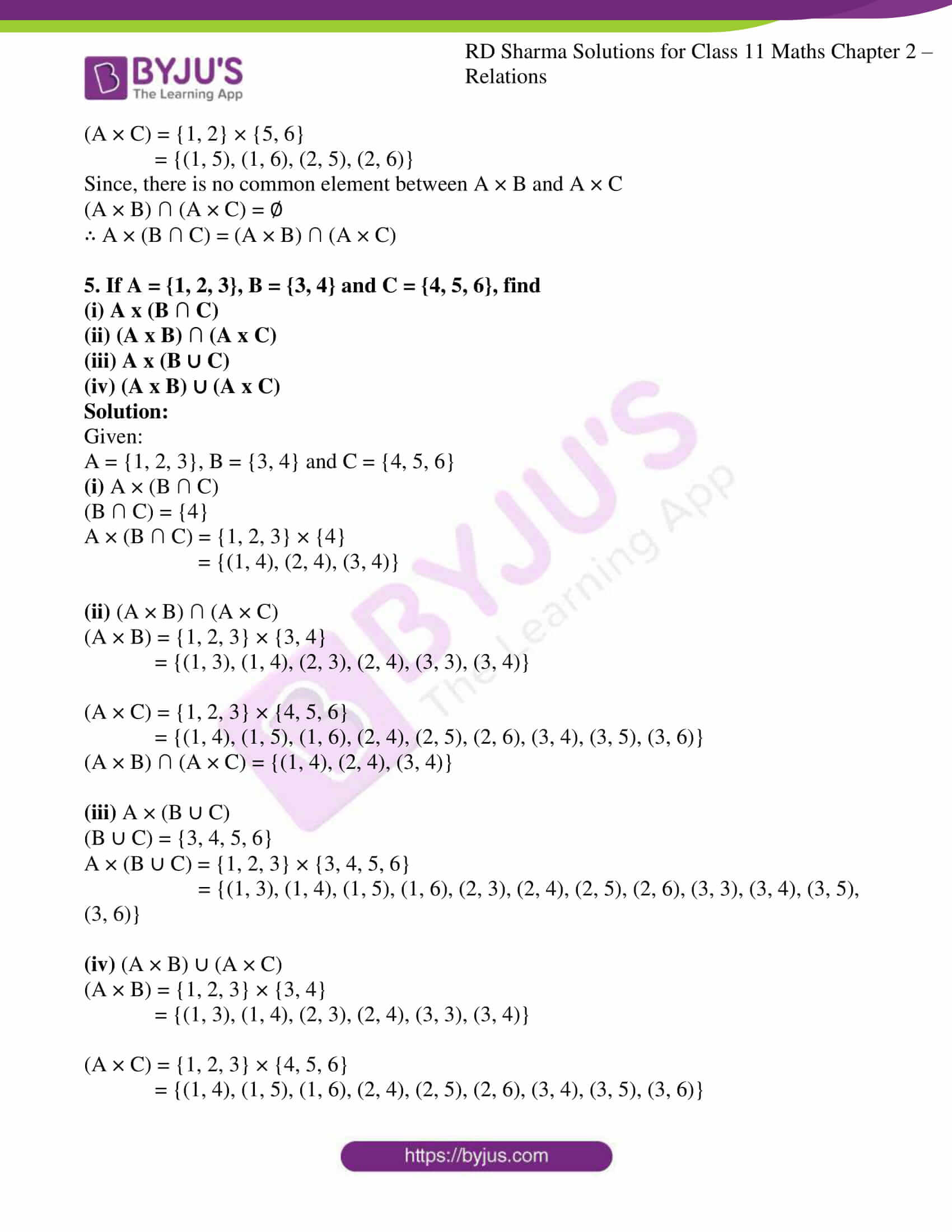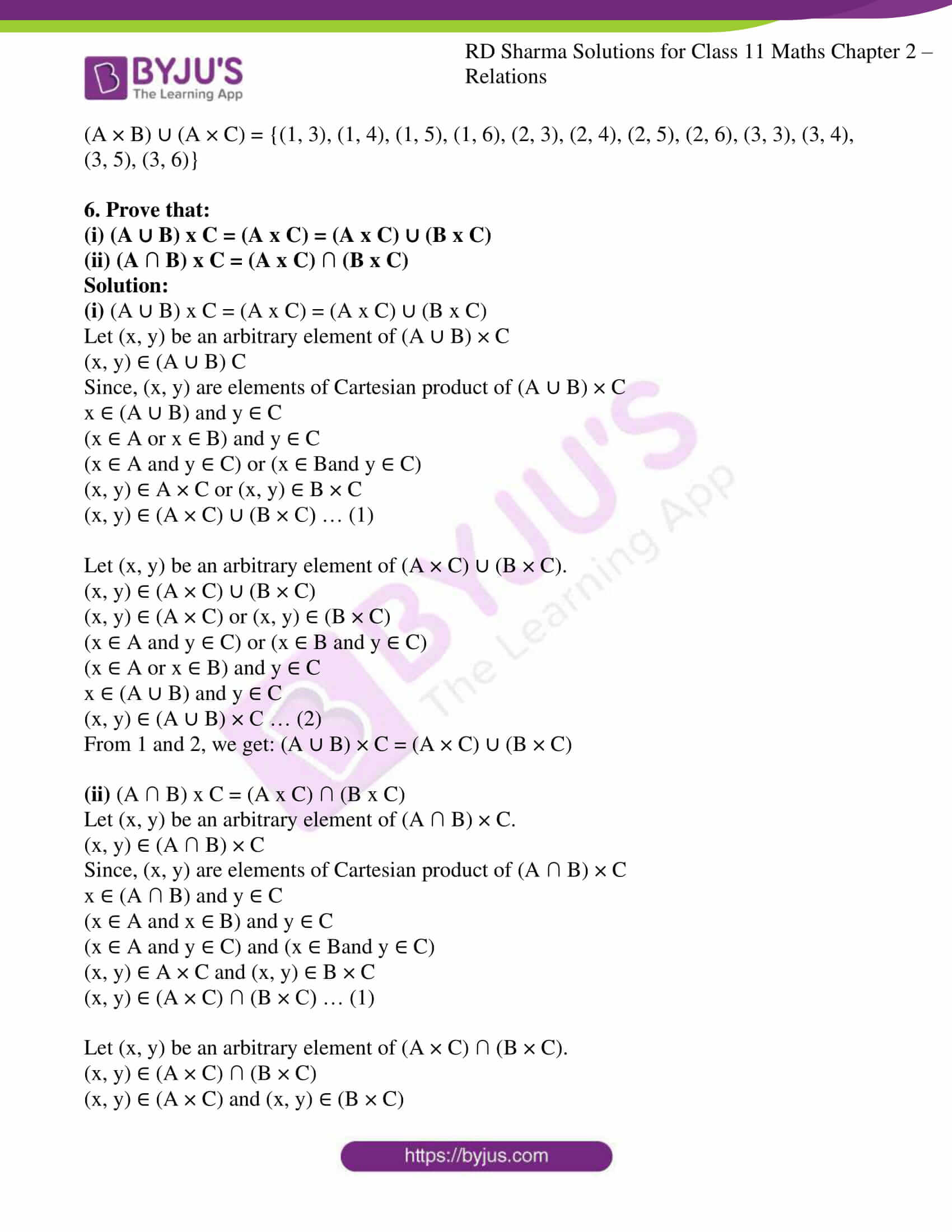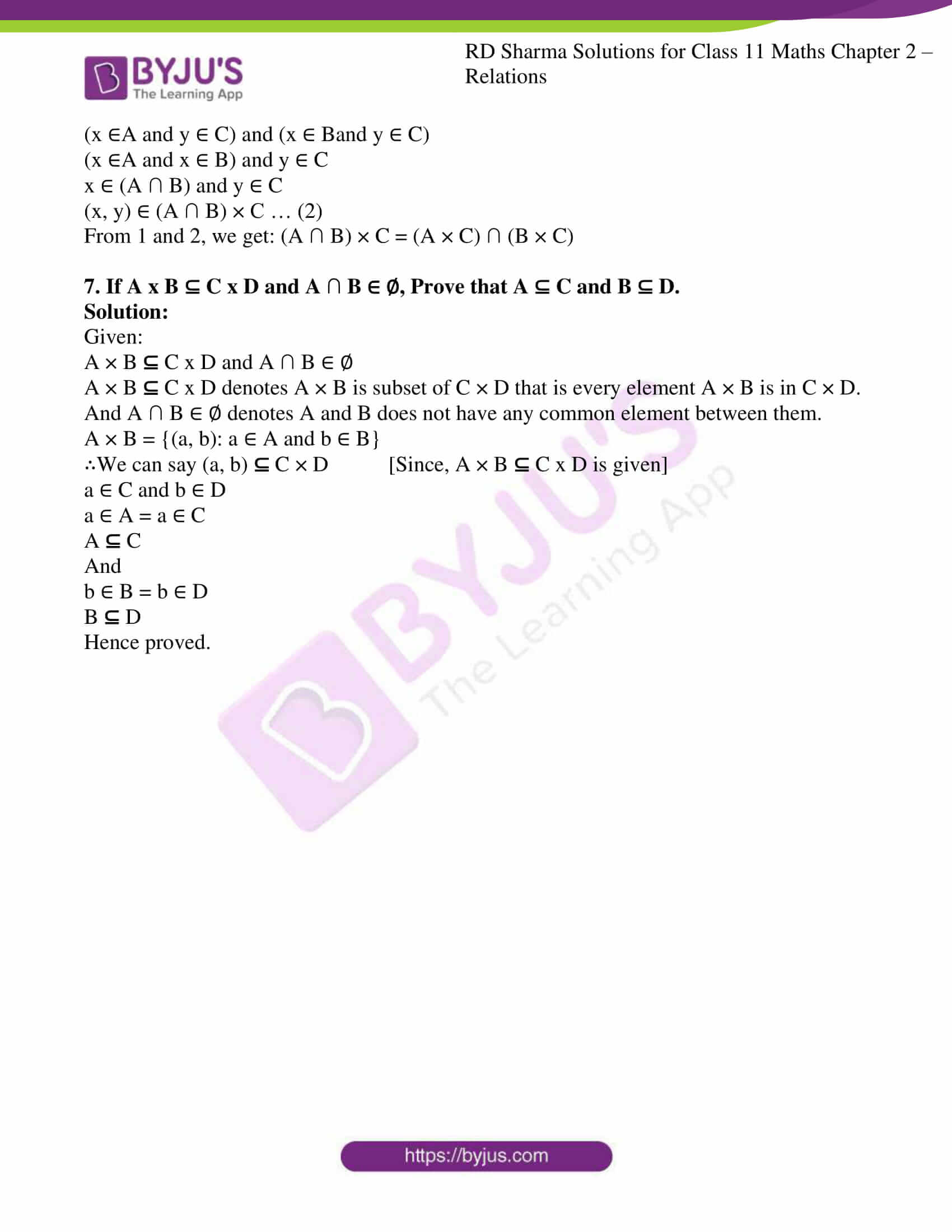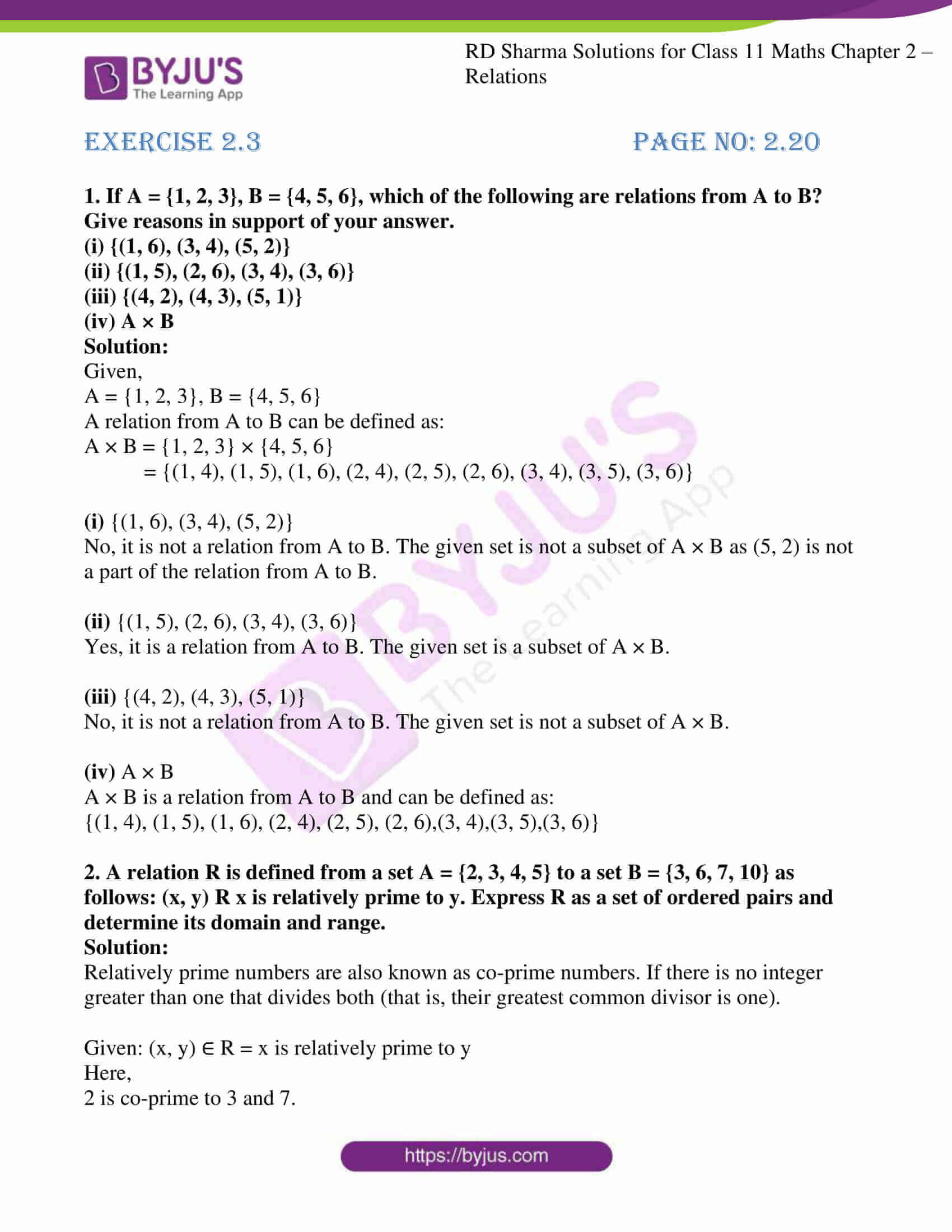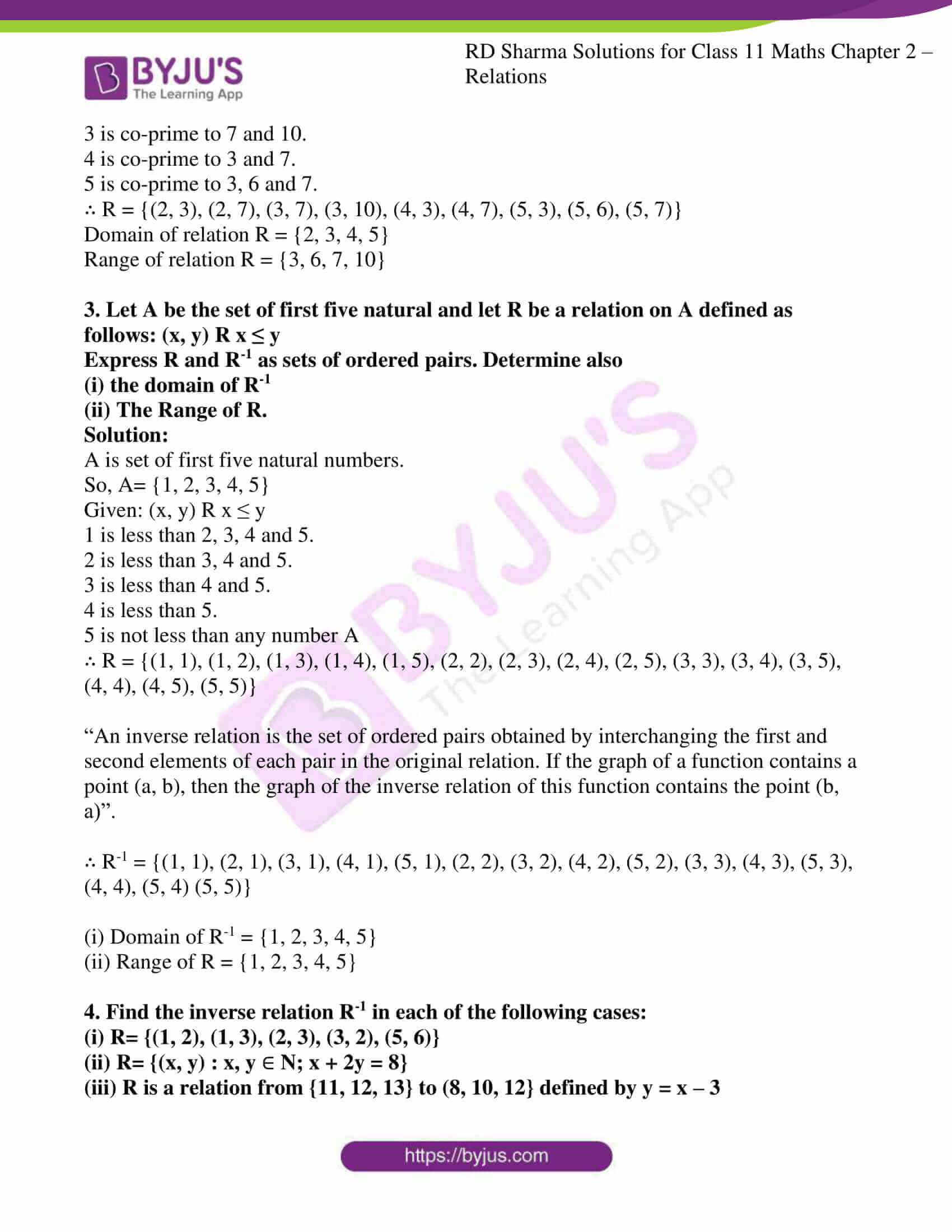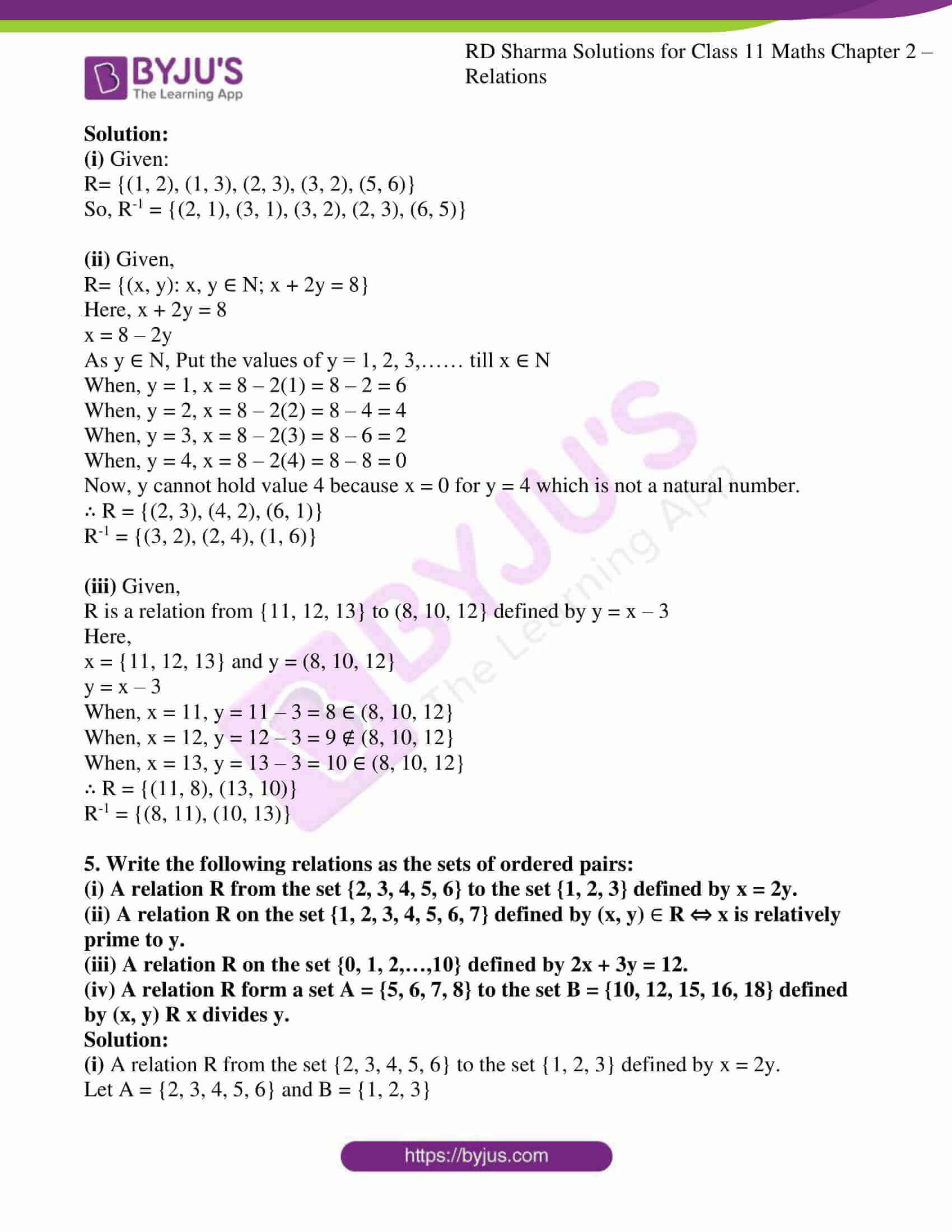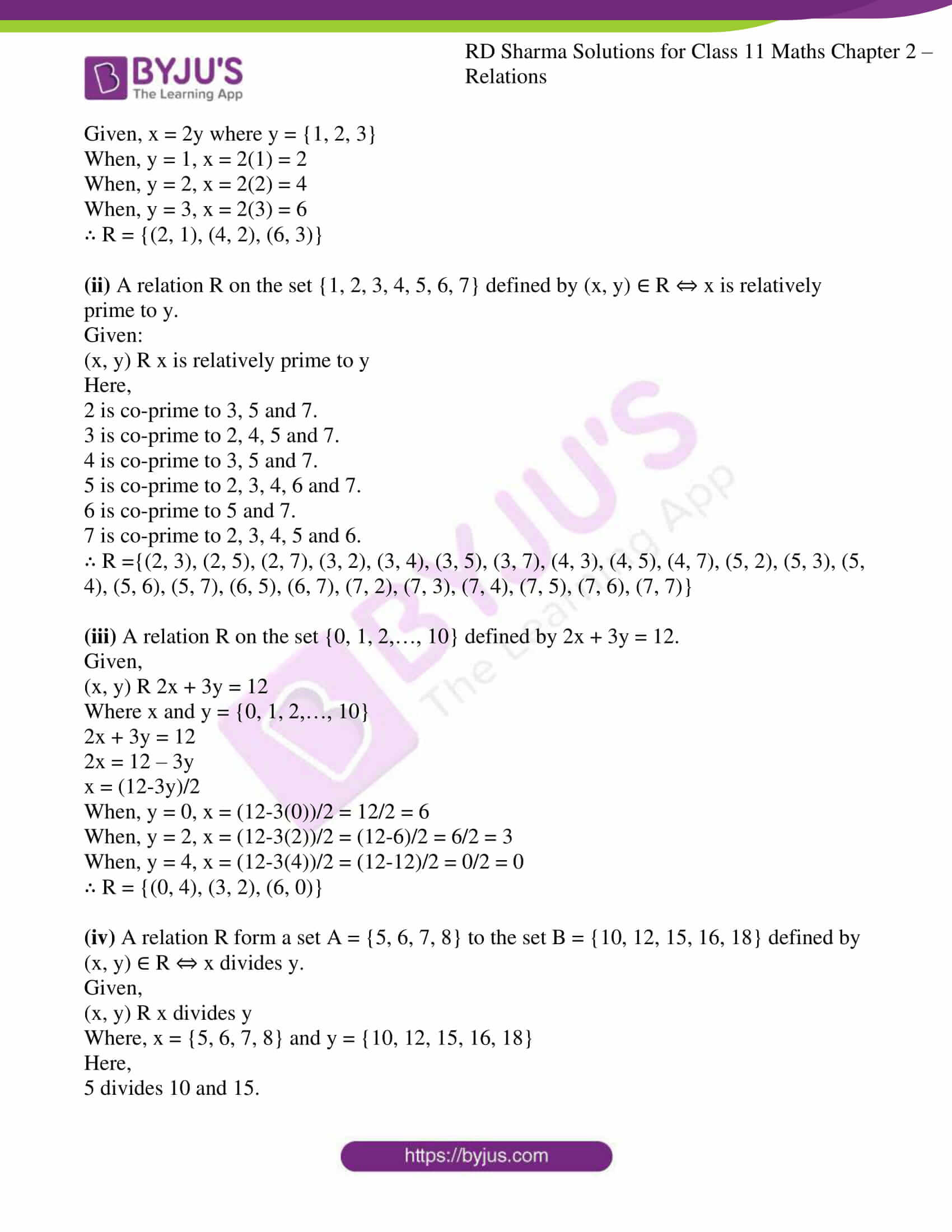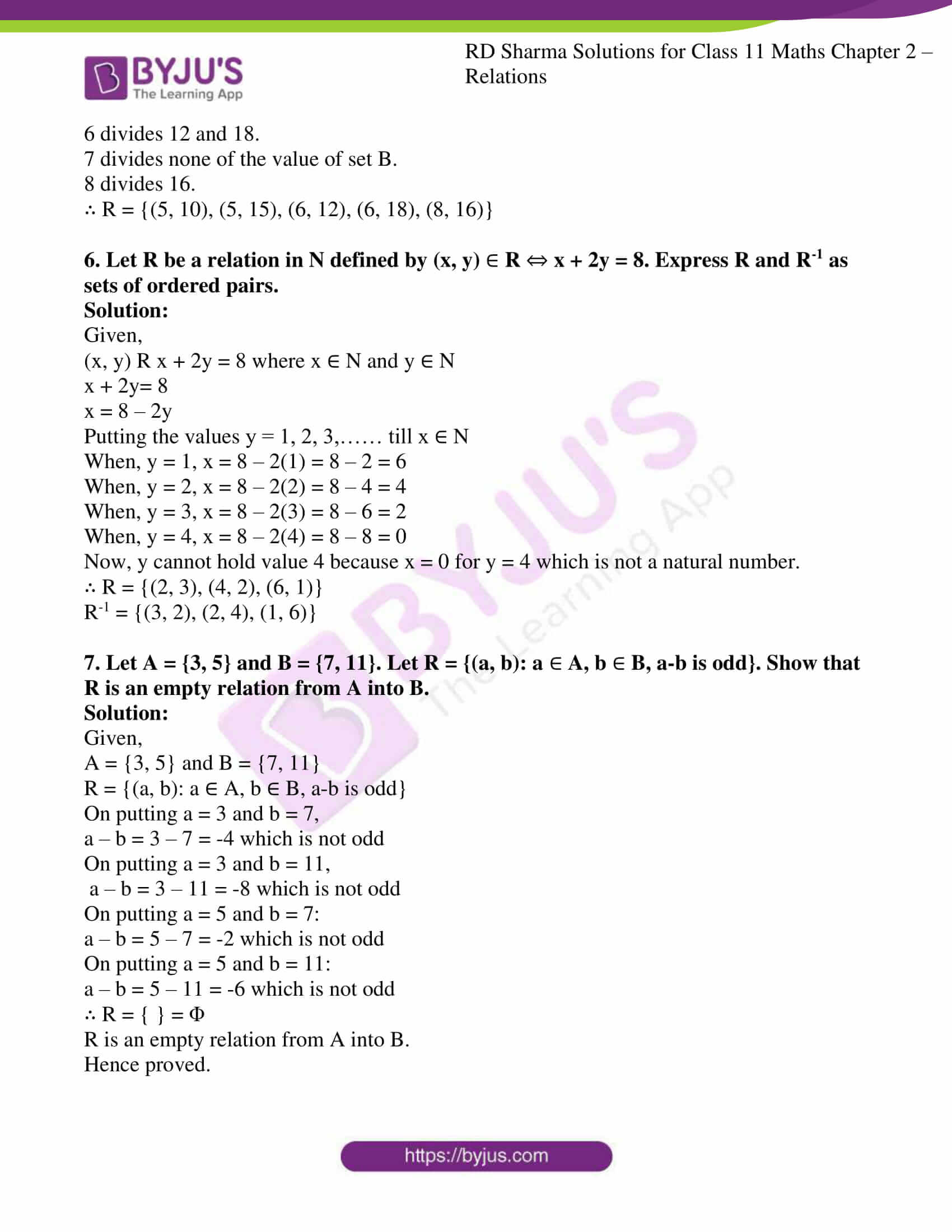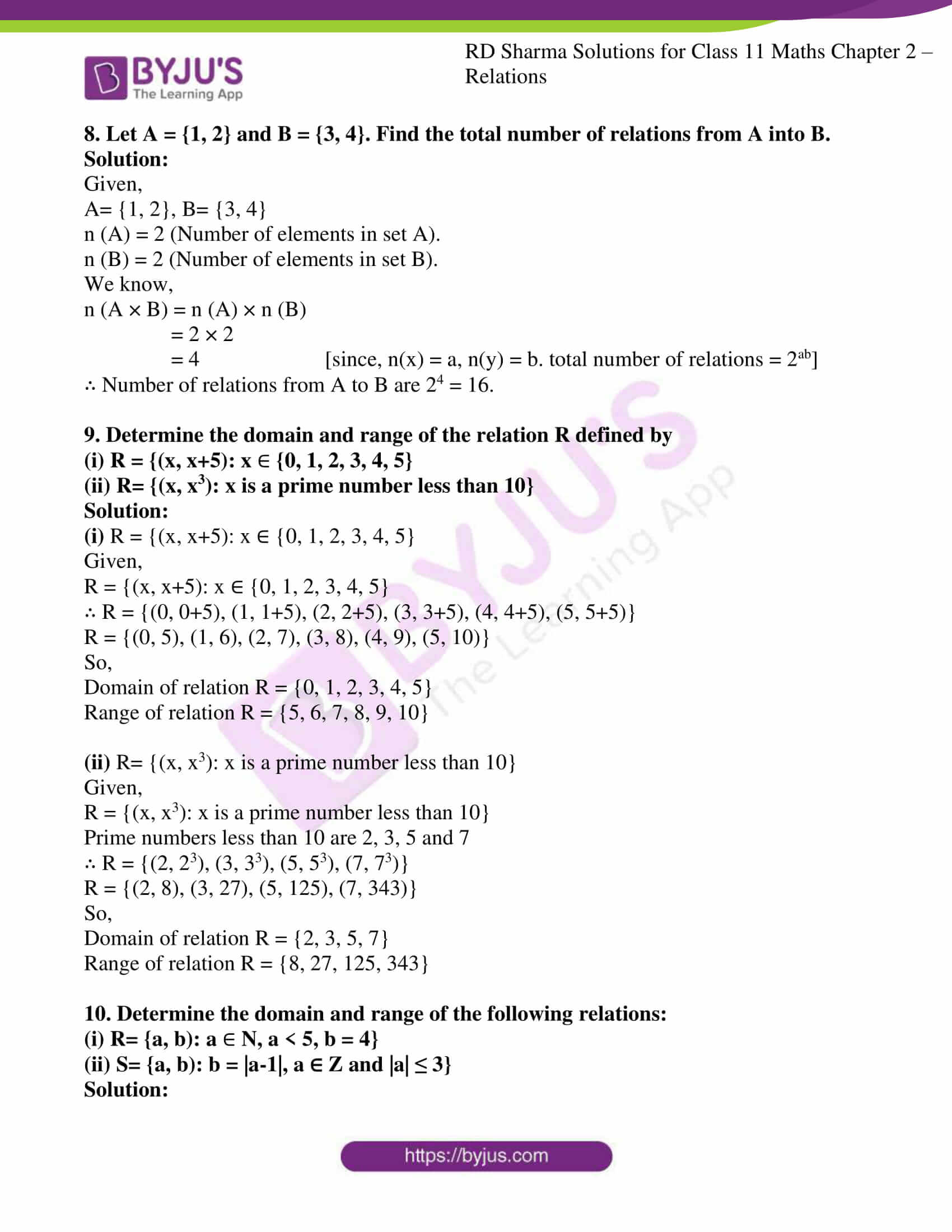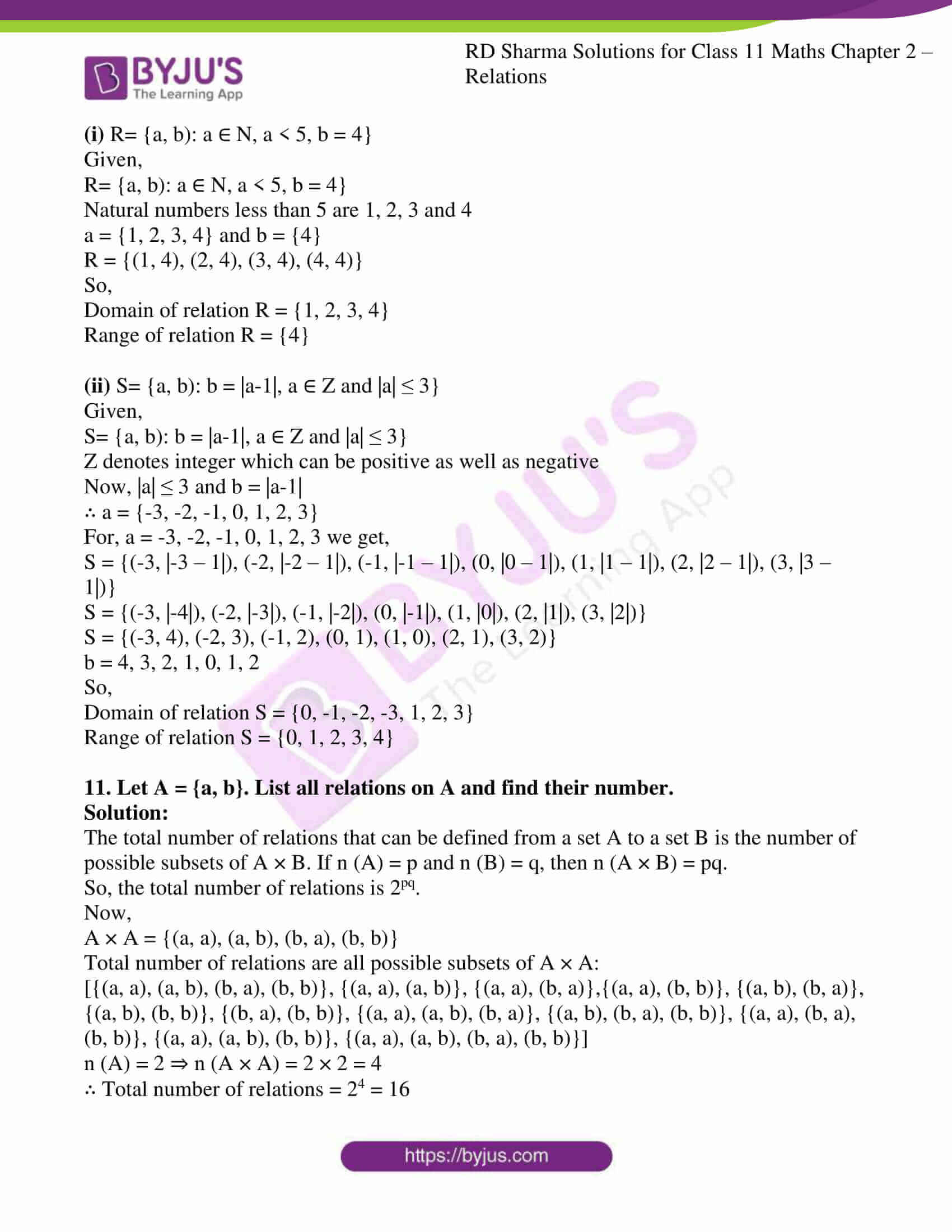### Access answers to RD Sharma Solutions for Class 11 Maths Chapter 2 – Relations

EXERCISE 2.1 PAGE NO: 2.8

(1) (i) If (a/3 + 1, b – 2/3) = (5/3, 1/3), find the values of a and b.

(ii) If (x + 1, 1) = (3y, y – 1), find the values of x and y.

Solution:

Given:

(a/3 + 1, b – 2/3) = (5/3, 1/3)

By the definition of equality of ordered pairs,

Let us solve for a and b

a/3 + 1 = 5/3 and b – 2/3 = 1/3

a/3 = 5/3 – 1 and b = 1/3 + 2/3

a/3 = (5-3)/3 and b = (1+2)/3

a/3 = 2/3 and b = 3/3

a = 2(3)/3 and b = 1

a = 2 and b = 1

∴ Values of a and b are, a = 2 and b = 1

(ii) If (x + 1, 1) = (3y, y – 1), find the values of x and y.

Given:

(x + 1, 1) = (3y, y – 1)

By the definition of equality of ordered pairs,

Let us solve for x and y

x + 1 = 3y and 1 = y – 1

x = 3y – 1 and y = 1 + 1

x = 3y – 1 and y = 2

Since, y = 2 we can substitute in

x = 3y – 1

= 3(2) – 1

= 6 – 1

= 5

∴ Values of x and y are, x = 5 and y = 2

2. If the ordered pairs (x, – 1) and (5, y) belong to the set {(a, b): b = 2a – 3}, find the values of x and y.

Solution:

Given:

The ordered pairs (x, – 1) and (5, y) belong to the set {(a, b): b = 2a – 3}

Solving for first order pair

(x, – 1) = {(a, b): b = 2a – 3}

x = a and -1 = b

By taking b = 2a – 3

If b = – 1 then 2a = – 1 + 3

= 2

a = 2/2

= 1

So, a = 1

Since x = a, x = 1

Similarly, solving for second order pair

(5, y) = {(a, b): b = 2a – 3}

5 = a and y = b

By taking b = 2a – 3

If a = 5 then b = 2×5 – 3

= 10 – 3

= 7

So, b = 7

Since y = b, y = 7

∴ Values of x and y are, x = 1 and y = 7

3. If a ∈ {- 1, 2, 3, 4, 5} and b ∈ {0, 3, 6}, write the set of all ordered pairs (a, b) such that a + b = 5.

Solution:

Given: a ∈ {- 1, 2, 3, 4, 5} and b ∈ {0, 3, 6},

To find: the ordered pair (a, b) such that a + b = 5

Then the ordered pair (a, b) such that a + b = 5 are as follows

(a, b) ∈ {(- 1, 6), (2, 3), (5, 0)}

4. If a ∈ {2, 4, 6, 9} and b ∈ {4, 6, 18, 27}, then form the set of all ordered pairs (a, b) such that a divides b and a<b.

Solution:

Given:

a ∈ {2, 4, 6, 9} and b ∈{4, 6, 18, 27}

Here,

2 divides 4, 6, 18 and is also less than all of them

4 divides 4 and is also less than none of them

6 divides 6, 18 and is less than 18 only

9 divides 18, 27 and is less than all of them

∴ Ordered pairs (a, b) are (2, 4), (2, 6), (2, 18), (6, 18), (9, 18) and (9, 27)

5. If A = {1, 2} and B = {1, 3}, find A x B and B x A.

Solution:

Given:

A = {1, 2} and B = {1, 3}

A × B = {1, 2} × {1, 3}

= {(1, 1), (1, 3), (2, 1), (2, 3)}

B × A = {1, 3} × {1, 2}

= {(1, 1), (1, 2), (3, 1), (3, 2)}

6. Let A = {1, 2, 3} and B = {3, 4}. Find A x B and show it graphically

Solution:

Given:

A = {1, 2, 3} and B = {3, 4}

A x B = {1, 2, 3} × {3, 4}

= {(1, 3), (1, 4), (2, 3), (2, 4), (3, 3), (3, 4)}

Steps to follow to represent A × B graphically,

Step 1: One horizontal and one vertical axis should be drawn

Step 2: Element of set A should be represented in horizontal axis and on vertical axis elements of set B should be represented

Step 3: Draw dotted lines perpendicular to horizontal and vertical axes through the elements of set A and B

Step 4: Point of intersection of these perpendicular represents A × B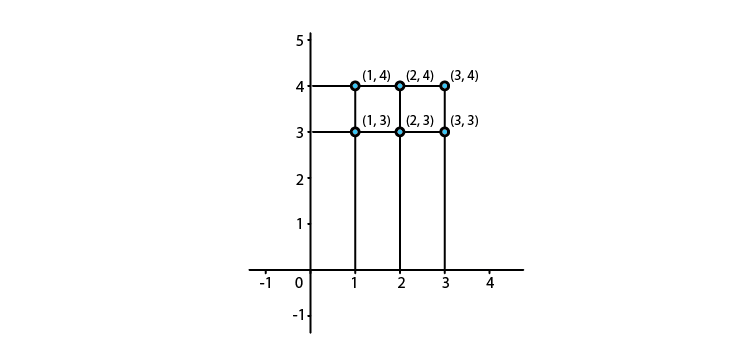7. If A = {1, 2, 3} and B = {2, 4}, what are A x B, B x A, A x A, B x B, and (A x B) ∩ (B x A)?

Solution:

Given:

A = {1, 2, 3} and B = {2, 4}

Now let us find: A × B, B × A, A × A, (A × B) ∩ (B × A)

A × B = {1, 2, 3} × {2, 4}

= {(1, 2), (1, 4), (2, 2), (2, 4), (3, 2), (3, 4)}

B × A = {2, 4} × {1, 2, 3}

= {(2, 1), (2, 2), (2, 3), (4, 1), (4, 2), (4, 3)}

A × A = {1, 2, 3} × {1, 2, 3}

= {(1, 1), (1, 2), (1, 3), (2, 1), (2, 2), (2, 3), (3, 1), (3, 2), (3, 3)}

B × B = {2, 4} × {2, 4}

= {(2, 2), (2, 4), (4, 2), (4, 4)}

Intersection of two sets represents common elements of both the sets

So,

(A × B) ∩ (B × A) = {(2, 2)}

EXERCISE 2.2 PAGE NO: 2.12

1. Given A = {1, 2, 3}, B = {3, 4}, C = {4, 5, 6}, find (A x B) ∩ (B x C).

Solution:

Given:

A = {1, 2, 3}, B = {3, 4} and C = {4, 5, 6}

Let us find: (A × B) ∩ (B × C)

(A × B) = {1, 2, 3} × {3, 4}

= {(1, 3), (1, 4), (2, 3), (2, 4), (3, 3), (3, 4)}

(B × C) = {3, 4} × {4, 5, 6}

= {(3, 4), (3, 5), (3, 6), (4, 4), (4, 5), (4, 6)}

∴ (A × B) ∩ (B × C) = {(3, 4)}

2. If A = {2, 3}, B = {4, 5}, C = {5, 6} find A x (B ∪ C), (A x B) ∪ (A x C).

Solution:

Given: A = {2, 3}, B = {4, 5} and C = {5, 6}

Let us find: A x (B ∪ C) and (A x B) ∪ (A x C)

(B ∪ C) = {4, 5, 6}

A × (B ∪ C) = {2, 3} × {4, 5, 6}

= {(2, 4), (2, 5), (2, 6), (3, 4), (3, 5), (3, 6)}

(A × B) = {2, 3} × {4, 5}

= {(2, 4), (2, 5), (3, 4), (3, 5)}

(A × C) = {2, 3} × {5, 6}

= {(2, 5), (2, 6), (3, 5), (3, 6)}

∴ (A × B) ∪ (A × C) = {(2, 4), (2, 5), (2, 6), (3, 4), (3, 5), (3, 6)}

A × (B ∪ C) = {(2, 4), (2, 5), (2, 6), (3, 4), (3, 5), (3, 6)}

3. If A = {1, 2, 3}, B = {4}, C = {5}, then verify that:
(i) A x (B
C) = (A x B)  (A x C)
(ii) A x (B ∩ C) = (A x B) ∩ (A x C)
(iii) A x (B – C) = (A x B) – (A x C)

Solution:

Given:

A = {1, 2, 3}, B = {4} and C = {5}

(i) A × (B ∪ C) = (A × B) ∪ (A × C)

Let us consider LHS: (B ∪ C)

(B ∪ C) = {4, 5}

A × (B ∪ C) = {1, 2, 3} × {4, 5}

= {(1, 4), (1, 5), (2, 4), (2, 5), (3, 4), (3, 5)}

Now, RHS

(A × B) = {1, 2, 3} × {4}

= {(1, 4), (2, 4), (3, 4)}

(A × C) = {1, 2, 3} × {5}

= {(1, 5), (2, 5), (3, 5)}

(A × B) ∪ (A × C) = {(1, 4), (2, 4), (3, 4), (1, 5), (2, 5), (3, 5)}

∴ LHS = RHS

(ii) A × (B ∩ C) = (A × B) ∩ (A × C)

Let us consider LHS: (B ∩ C)

(B ∩ C) = ∅ (No common element)

A × (B ∩ C) = {1, 2, 3} × ∅

= ∅

Now, RHS

(A × B) = {1, 2, 3} × {4}

= {(1, 4), (2, 4), (3, 4)}

(A × C) = {1, 2, 3} × {5}

= {(1, 5), (2, 5), (3, 5)}

(A × B) ∩ (A × C) = ∅

∴ LHS = RHS

(iii) A × (B − C) = (A × B) − (A × C)

Let us consider LHS: (B − C)

(B − C) = ∅

A × (B − C) = {1, 2, 3} × ∅

= ∅

Now, RHS

(A × B) = {1, 2, 3} × {4}

= {(1, 4), (2, 4), (3, 4)}

(A × C) = {1, 2, 3} × {5}

= {(1, 5), (2, 5), (3, 5)}

(A × B) − (A × C) = ∅

∴ LHS = RHS

4. Let A = {1, 2}, B = {1, 2, 3, 4}, C = {5, 6} and D = {5, 6, 7, 8}. Verify that:
(i) A x C ⊂ B x D
(ii) A x (B ∩ C) = (A x B) ∩ (A x C)

Solution:

Given:

A = {1, 2}, B = {1, 2, 3, 4}, C = {5, 6} and D = {5, 6, 7, 8}

(i) A x C ⊂ B x D

Let us consider LHS A x C

A × C = {1, 2} × {5, 6}

= {(1, 5), (1, 6), (2, 5), (2, 6)}

Now, RHS

B × D = {1, 2, 3, 4} × {5, 6, 7, 8}

= {(1, 5), (1, 6), (1, 7), (1, 8), (2, 5), (2, 6), (2, 7), (2, 8), (3, 5), (3, 6), (3, 7), (3, 8), (4, 5), (4, 6), (4, 7), (4, 8)}

Since, all elements of A × C is in B × D.

∴We can say A × C ⊂ B × D

(ii) A × (B ∩ C) = (A × B) ∩ (A × C)

Let us consider LHS A × (B ∩ C)

(B ∩ C) = ∅

A × (B ∩ C) = {1, 2} × ∅

= ∅

Now, RHS

(A × B) = {1, 2} × {1, 2, 3, 4}

= {(1, 1), (1, 2), (1, 3), (1, 4), (2, 1), (2, 2), (2, 3), (2, 4)}

(A × C) = {1, 2} × {5, 6}

= {(1, 5), (1, 6), (2, 5), (2, 6)}

Since, there is no common element between A × B and A × C

(A × B) ∩ (A × C) = ∅

∴ A × (B ∩ C) = (A × B) ∩ (A × C)

5. If A = {1, 2, 3}, B = {3, 4} and C = {4, 5, 6}, find
(i) A x (B ∩ C)
(ii) (A x B) ∩ (A x C)
(iii) A x (B ∪ C)
(iv) (A x B) ∪ (A x C)

Solution:

Given:

A = {1, 2, 3}, B = {3, 4} and C = {4, 5, 6}

(i) A × (B ∩ C)

(B ∩ C) = {4}

A × (B ∩ C) = {1, 2, 3} × {4}

= {(1, 4), (2, 4), (3, 4)}

(ii) (A × B) ∩ (A × C)

(A × B) = {1, 2, 3} × {3, 4}

= {(1, 3), (1, 4), (2, 3), (2, 4), (3, 3), (3, 4)}

(A × C) = {1, 2, 3} × {4, 5, 6}

= {(1, 4), (1, 5), (1, 6), (2, 4), (2, 5), (2, 6), (3, 4), (3, 5), (3, 6)}

(A × B) ∩ (A × C) = {(1, 4), (2, 4), (3, 4)}

(iii) A × (B ∪ C)

(B ∪ C) = {3, 4, 5, 6}

A × (B ∪ C) = {1, 2, 3} × {3, 4, 5, 6}

= {(1, 3), (1, 4), (1, 5), (1, 6), (2, 3), (2, 4), (2, 5), (2, 6), (3, 3), (3, 4), (3, 5), (3, 6)}

(iv) (A × B) ∪ (A × C)

(A × B) = {1, 2, 3} × {3, 4}

= {(1, 3), (1, 4), (2, 3), (2, 4), (3, 3), (3, 4)}

(A × C) = {1, 2, 3} × {4, 5, 6}

= {(1, 4), (1, 5), (1, 6), (2, 4), (2, 5), (2, 6), (3, 4), (3, 5), (3, 6)}

(A × B) ∪ (A × C) = {(1, 3), (1, 4), (1, 5), (1, 6), (2, 3), (2, 4), (2, 5), (2, 6), (3, 3), (3, 4), (3, 5), (3, 6)}

6. Prove that:
(i) (A ∪ B) x C = (A x C) = (A x C) ∪ (B x C)

(ii) (A ∩ B) x C = (A x C) ∩ (B x C)

Solution:

(i) (A ∪ B) x C = (A x C) = (A x C) ∪ (B x C)

Let (x, y) be an arbitrary element of (A ∪ B) × C

(x, y) ∈ (A ∪ B) C

Since, (x, y) are elements of Cartesian product of (A ∪ B) × C

x ∈ (A ∪ B) and y ∈ C

(x ∈ A or x ∈ B) and y ∈ C

(x ∈ A and y ∈ C) or (x ∈ Band y ∈ C)

(x, y) ∈ A × C or (x, y) ∈ B × C

(x, y) ∈ (A × C) ∪ (B × C) … (1)

Let (x, y) be an arbitrary element of (A × C) ∪ (B × C).

(x, y) ∈ (A × C) ∪ (B × C)

(x, y) ∈ (A × C) or (x, y) ∈ (B × C)

(x ∈ A and y ∈ C) or (x ∈ B and y ∈ C)

(x ∈ A or x ∈ B) and y ∈ C

x ∈ (A ∪ B) and y ∈ C

(x, y) ∈ (A ∪ B) × C … (2)

From 1 and 2, we get: (A ∪ B) × C = (A × C) ∪ (B × C)

(ii) (A ∩ B) x C = (A x C) ∩ (B x C)

Let (x, y) be an arbitrary element of (A ∩ B) × C.

(x, y) ∈ (A ∩ B) × C

Since, (x, y) are elements of Cartesian product of (A ∩ B) × C

x ∈ (A ∩ B) and y ∈ C

(x ∈ A and x ∈ B) and y ∈ C

(x ∈ A and y ∈ C) and (x ∈ Band y ∈ C)

(x, y) ∈ A × C and (x, y) ∈ B × C

(x, y) ∈ (A × C) ∩ (B × C) … (1)

Let (x, y) be an arbitrary element of (A × C) ∩ (B × C).

(x, y) ∈ (A × C) ∩ (B × C)

(x, y) ∈ (A × C) and (x, y) ∈ (B × C)

(x ∈A and y ∈ C) and (x ∈ Band y ∈ C)

(x ∈A and x ∈ B) and y ∈ C

x ∈ (A ∩ B) and y ∈ C

(x, y) ∈ (A ∩ B) × C … (2)

From 1 and 2, we get: (A ∩ B) × C = (A × C) ∩ (B × C)

7. If A x B ⊆ C x D and A ∩ B ∈ ∅, Prove that A ⊆ C and B ⊆ D.

Solution:

Given:

A × B  C x D and A ∩ B ∈ ∅

A × B  C x D denotes A × B is subset of C × D that is every element A × B is in C × D.

And A ∩ B ∈ ∅ denotes A and B does not have any common element between them.

A × B = {(a, b): a ∈ A and b ∈ B}

∴We can say (a, b)  C × D [Since, A × B  C x D is given]

a ∈ C and b ∈ D

a ∈ A = a ∈ C

A  C

And

b ∈ B = b ∈ D

B  D

Hence proved.

EXERCISE 2.3 PAGE NO: 2.20

1. If A = {1, 2, 3}, B = {4, 5, 6}, which of the following are relations from A to B?

(i) {(1, 6), (3, 4), (5, 2)}

(ii) {(1, 5), (2, 6), (3, 4), (3, 6)}

(iii) {(4, 2), (4, 3), (5, 1)}

(iv) A × B

Solution:

Given,

A = {1, 2, 3}, B = {4, 5, 6}

A relation from A to B can be defined as:

A × B = {1, 2, 3} × {4, 5, 6}

= {(1, 4), (1, 5), (1, 6), (2, 4), (2, 5), (2, 6), (3, 4), (3, 5), (3, 6)}

(i) {(1, 6), (3, 4), (5, 2)}

No, it is not a relation from A to B. The given set is not a subset of A × B as (5, 2) is not a part of the relation from A to B.

(ii) {(1, 5), (2, 6), (3, 4), (3, 6)}

Yes, it is a relation from A to B. The given set is a subset of A × B.

(iii) {(4, 2), (4, 3), (5, 1)}

No, it is not a relation from A to B. The given set is not a subset of A × B.

(iv) A × B

A × B is a relation from A to B and can be defined as:

{(1, 4), (1, 5), (1, 6), (2, 4), (2, 5), (2, 6),(3, 4),(3, 5),(3, 6)}

2. A relation R is defined from a set A = {2, 3, 4, 5} to a set B = {3, 6, 7, 10} as follows: (x, y) R x is relatively prime to y. Express R as a set of ordered pairs and determine its domain and range.

Solution:

Relatively prime numbers are also known as co-prime numbers. If there is no integer greater than one that divides both (that is, their greatest common divisor is one).

Given: (x, y) ∈ R = x is relatively prime to y

Here,

2 is co-prime to 3 and 7.

3 is co-prime to 7 and 10.

4 is co-prime to 3 and 7.

5 is co-prime to 3, 6 and 7.

∴ R = {(2, 3), (2, 7), (3, 7), (3, 10), (4, 3), (4, 7), (5, 3), (5, 6), (5, 7)}

Domain of relation R = {2, 3, 4, 5}

Range of relation R = {3, 6, 7, 10}

3. Let A be the set of first five natural and let R be a relation on A defined as follows: (x, y) R x ≤ y
Express R and R-1 as sets of ordered pairs. Determine also
(i) the domain of R‑1
(ii) The Range of R.

Solution:

A is set of first five natural numbers.

So, A= {1, 2, 3, 4, 5}

Given: (x, y) R x ≤ y

1 is less than 2, 3, 4 and 5.

2 is less than 3, 4 and 5.

3 is less than 4 and 5.

4 is less than 5.

5 is not less than any number A

∴ R = {(1, 1), (1, 2), (1, 3), (1, 4), (1, 5), (2, 2), (2, 3), (2, 4), (2, 5), (3, 3), (3, 4), (3, 5), (4, 4), (4, 5), (5, 5)}

“An inverse relation is the set of ordered pairs obtained by interchanging the first and second elements of each pair in the original relation. If the graph of a function contains a point (a, b), then the graph of the inverse relation of this function contains the point (b, a)”.

∴ R-1 = {(1, 1), (2, 1), (3, 1), (4, 1), (5, 1), (2, 2), (3, 2), (4, 2), (5, 2), (3, 3), (4, 3), (5, 3), (4, 4), (5, 4) (5, 5)}

(i) Domain of R‑1 = {1, 2, 3, 4, 5}

(ii) Range of R = {1, 2, 3, 4, 5}

4. Find the inverse relation R-1 in each of the following cases:
(i) R= {(1, 2), (1, 3), (2, 3), (3, 2), (5, 6)}
(ii) R= {(x, y) : x, y
N; x + 2y = 8}
(iii) R is a relation from {11, 12, 13} to (8, 10, 12} defined by y = x – 3

Solution:

(i) Given:

R= {(1, 2), (1, 3), (2, 3), (3, 2), (5, 6)}

So, R‑1 = {(2, 1), (3, 1), (3, 2), (2, 3), (6, 5)}

(ii) Given,

R= {(x, y): x, y ∈ N; x + 2y = 8}

Here, x + 2y = 8

x = 8 – 2y

As y ∈ N, Put the values of y = 1, 2, 3,…… till x ∈ N

When, y = 1, x = 8 – 2(1) = 8 – 2 = 6

When, y = 2, x = 8 – 2(2) = 8 – 4 = 4

When, y = 3, x = 8 – 2(3) = 8 – 6 = 2

When, y = 4, x = 8 – 2(4) = 8 – 8 = 0

Now, y cannot hold value 4 because x = 0 for y = 4 which is not a natural number.

∴ R = {(2, 3), (4, 2), (6, 1)}

R‑1 = {(3, 2), (2, 4), (1, 6)}

(iii) Given,

R is a relation from {11, 12, 13} to (8, 10, 12} defined by y = x – 3

Here,

x = {11, 12, 13} and y = (8, 10, 12}

y = x – 3

When, x = 11, y = 11 – 3 = 8 ∈ (8, 10, 12}

When, x = 12, y = 12 – 3 = 9 ∉ (8, 10, 12}

When, x = 13, y = 13 – 3 = 10 ∈ (8, 10, 12}

∴ R = {(11, 8), (13, 10)}

R‑1 = {(8, 11), (10, 13)}

5. Write the following relations as the sets of ordered pairs:
(i) A relation R from the set {2, 3, 4, 5, 6} to the set {1, 2, 3} defined by x = 2y.

(ii) A relation R on the set {1, 2, 3, 4, 5, 6, 7} defined by (x, y)  R ⇔ x is relatively prime to y.

(iii) A relation R on the set {0, 1, 2,…,10} defined by 2x + 3y = 12.

(iv) A relation R form a set A = {5, 6, 7, 8} to the set B = {10, 12, 15, 16, 18} defined by (x, y) R x divides y.

Solution:

(i) A relation R from the set {2, 3, 4, 5, 6} to the set {1, 2, 3} defined by x = 2y.

Let A = {2, 3, 4, 5, 6} and B = {1, 2, 3}

Given, x = 2y where y = {1, 2, 3}

When, y = 1, x = 2(1) = 2

When, y = 2, x = 2(2) = 4

When, y = 3, x = 2(3) = 6

∴ R = {(2, 1), (4, 2), (6, 3)}

(ii) A relation R on the set {1, 2, 3, 4, 5, 6, 7} defined by (x, y) ∈ R ⇔ x is relatively prime to y.

Given:

(x, y) R x is relatively prime to y

Here,

2 is co-prime to 3, 5 and 7.

3 is co-prime to 2, 4, 5 and 7.

4 is co-prime to 3, 5 and 7.

5 is co-prime to 2, 3, 4, 6 and 7.

6 is co-prime to 5 and 7.

7 is co-prime to 2, 3, 4, 5 and 6.

∴ R ={(2, 3), (2, 5), (2, 7), (3, 2), (3, 4), (3, 5), (3, 7), (4, 3), (4, 5), (4, 7), (5, 2), (5, 3), (5, 4), (5, 6), (5, 7), (6, 5), (6, 7), (7, 2), (7, 3), (7, 4), (7, 5), (7, 6), (7, 7)}

(iii) A relation R on the set {0, 1, 2,…, 10} defined by 2x + 3y = 12.

Given,

(x, y) R 2x + 3y = 12

Where x and y = {0, 1, 2,…, 10}

2x + 3y = 12

2x = 12 – 3y

x = (12-3y)/2

When, y = 0, x = (12-3(0))/2 = 12/2 = 6

When, y = 2, x = (12-3(2))/2 = (12-6)/2 = 6/2 = 3

When, y = 4, x = (12-3(4))/2 = (12-12)/2 = 0/2 = 0

∴ R = {(0, 4), (3, 2), (6, 0)}

(iv) A relation R form a set A = {5, 6, 7, 8} to the set B = {10, 12, 15, 16, 18} defined by (x, y) ∈ R ⇔ x divides y.

Given,

(x, y) R x divides y

Where, x = {5, 6, 7, 8} and y = {10, 12, 15, 16, 18}

Here,

5 divides 10 and 15.

6 divides 12 and 18.

7 divides none of the value of set B.

8 divides 16.

∴ R = {(5, 10), (5, 15), (6, 12), (6, 18), (8, 16)}

6. Let R be a relation in N defined by (x, y)  R  x + 2y = 8. Express R and R-1 as sets of ordered pairs.

Solution:

Given,

(x, y) R x + 2y = 8 where x ∈ N and y ∈ N

x + 2y= 8

x = 8 – 2y

Putting the values y = 1, 2, 3,…… till x ∈ N

When, y = 1, x = 8 – 2(1) = 8 – 2 = 6

When, y = 2, x = 8 – 2(2) = 8 – 4 = 4

When, y = 3, x = 8 – 2(3) = 8 – 6 = 2

When, y = 4, x = 8 – 2(4) = 8 – 8 = 0

Now, y cannot hold value 4 because x = 0 for y = 4 which is not a natural number.

∴ R = {(2, 3), (4, 2), (6, 1)}

R‑1 = {(3, 2), (2, 4), (1, 6)}

7. Let A = {3, 5} and B = {7, 11}. Let R = {(a, b): a  A, b  B, a-b is odd}. Show that R is an empty relation from A into B.

Solution:

Given,

A = {3, 5} and B = {7, 11}

R = {(a, b): a ∈ A, b ∈ B, a-b is odd}

On putting a = 3 and b = 7,

a – b = 3 – 7 = -4 which is not odd

On putting a = 3 and b = 11,

a – b = 3 – 11 = -8 which is not odd

On putting a = 5 and b = 7:

a – b = 5 – 7 = -2 which is not odd

On putting a = 5 and b = 11:

a – b = 5 – 11 = -6 which is not odd

∴ R = { } = Φ

R is an empty relation from A into B.

Hence proved.

8. Let A = {1, 2} and B = {3, 4}. Find the total number of relations from A into B.

Solution:

Given,

A= {1, 2}, B= {3, 4}

n (A) = 2 (Number of elements in set A).

n (B) = 2 (Number of elements in set B).

We know,

n (A × B) = n (A) × n (B)

= 2 × 2

= 4 [since, n(x) = a, n(y) = b. total number of relations = 2ab]

∴ Number of relations from A to B are 24 = 16.

9. Determine the domain and range of the relation R defined by
(i) R = {(x, x+5): x
{0, 1, 2, 3, 4, 5}

(ii) R= {(x, x3): x is a prime number less than 10}

Solution:

(i) R = {(x, x+5): x ∈ {0, 1, 2, 3, 4, 5}

Given,

R = {(x, x+5): x ∈ {0, 1, 2, 3, 4, 5}

∴ R = {(0, 0+5), (1, 1+5), (2, 2+5), (3, 3+5), (4, 4+5), (5, 5+5)}

R = {(0, 5), (1, 6), (2, 7), (3, 8), (4, 9), (5, 10)}

So,

Domain of relation R = {0, 1, 2, 3, 4, 5}

Range of relation R = {5, 6, 7, 8, 9, 10}

(ii) R= {(x, x3): x is a prime number less than 10}

Given,

R = {(x, x3): x is a prime number less than 10}

Prime numbers less than 10 are 2, 3, 5 and 7

∴ R = {(2, 23), (3, 33), (5, 53), (7, 73)}

R = {(2, 8), (3, 27), (5, 125), (7, 343)}

So,

Domain of relation R = {2, 3, 5, 7}

Range of relation R = {8, 27, 125, 343}

10. Determine the domain and range of the following relations:
(i) R= {a, b): a
N, a < 5, b = 4}

(ii) S= {a, b): b = |a-1|, a ∈ Z and |a| ≤ 3}

Solution:

(i) R= {a, b): a ∈ N, a < 5, b = 4}

Given,

R= {a, b): a ∈ N, a < 5, b = 4}

Natural numbers less than 5 are 1, 2, 3 and 4

a = {1, 2, 3, 4} and b = {4}

R = {(1, 4), (2, 4), (3, 4), (4, 4)}

So,

Domain of relation R = {1, 2, 3, 4}

Range of relation R = {4}

(ii) S= {a, b): b = |a-1|, a ∈ Z and |a| ≤ 3}

Given,

S= {a, b): b = |a-1|, a ∈ Z and |a| ≤ 3}

Z denotes integer which can be positive as well as negative

Now, |a| ≤ 3 and b = |a-1|

∴ a = {-3, -2, -1, 0, 1, 2, 3}

For, a = -3, -2, -1, 0, 1, 2, 3 we get,

S = {(-3, |-3 – 1|), (-2, |-2 – 1|), (-1, |-1 – 1|), (0, |0 – 1|), (1, |1 – 1|), (2, |2 – 1|), (3, |3 – 1|)}

S = {(-3, |-4|), (-2, |-3|), (-1, |-2|), (0, |-1|), (1, |0|), (2, |1|), (3, |2|)}

S = {(-3, 4), (-2, 3), (-1, 2), (0, 1), (1, 0), (2, 1), (3, 2)}

b = 4, 3, 2, 1, 0, 1, 2

So,

Domain of relation S = {0, -1, -2, -3, 1, 2, 3}

Range of relation S = {0, 1, 2, 3, 4}

11. Let A = {a, b}. List all relations on A and find their number.

Solution:

The total number of relations that can be defined from a set A to a set B is the number of possible subsets of A × B. If n (A) = p and n (B) = q, then n (A × B) = pq.

So, the total number of relations is 2pq.

Now,

A × A = {(a, a), (a, b), (b, a), (b, b)}

Total number of relations are all possible subsets of A × A:

[{(a, a), (a, b), (b, a), (b, b)}, {(a, a), (a, b)}, {(a, a), (b, a)},{(a, a), (b, b)}, {(a, b), (b, a)}, {(a, b), (b, b)}, {(b, a), (b, b)}, {(a, a), (a, b), (b, a)}, {(a, b), (b, a), (b, b)}, {(a, a), (b, a), (b, b)}, {(a, a), (a, b), (b, b)}, {(a, a), (a, b), (b, a), (b, b)}]

n (A) = 2 ⇒ n (A × A) = 2 × 2 = 4

∴ Total number of relations = 24 = 16

## Frequently Asked Questions on RD Sharma Solutions for Class 11 Maths Chapter 2

### Where can I get the accurate solution for RD Sharma Solution for Class 11 Maths Chapter 2?

At BYJU’S you can get the accurate solution in PDF format for RD Sharma Solution for Class 11 Maths Chapter 2. The RD Sharma Textbook Solutions for this chapter have been designed accurately by Mathematics experts at BYJU’S. All these solutions are provided by considering the new pattern of CBSE, so that students can get thorough knowledge for their exams.

### Is it necessary to solve each problem provided in the RD Sharma Solution for Class 11 Maths Chapter 2?

Yes. Because these questions are important from an exam perspective. These questions are solved by experts to help the students to crack exercise very easily. These solutions help students to familiarize themselves with the data handling. Solutions are available in PDF format on BYJU’S website.

### List out the concepts covered in RD Sharma Solution for Class 11 Maths Chapter 2?

The concepts are covered in RD Sharma Solution for Class 11 Maths Chapter 2 are provided below
Ordered pairs.
Cartesian product of sets.
Graphical representation of the cartesian product of sets.
The number of elements in the cartesian product of two sets.
Diagramatic representation of the cartesian product of two sets.
Relations.
Representation of a relation.
Domain and range of a relation.
The inverse of a relation.
By learning these concepts students will be able to solve questions on relations.